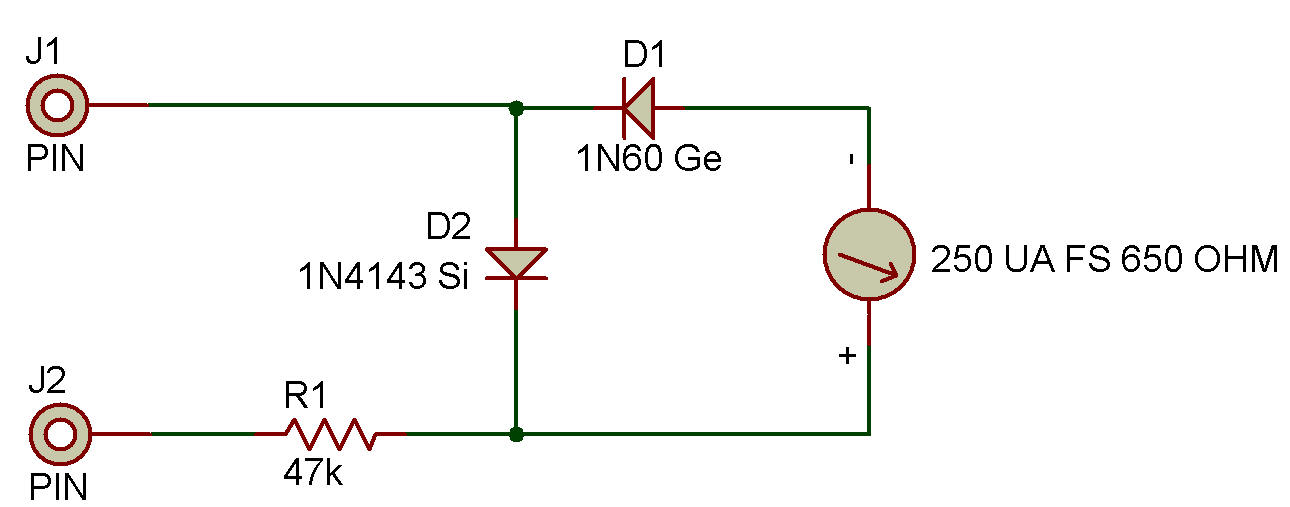Recherche personnalisé / personalised search

AC VoltmeterYou may have noticed that we called Project "DC voltmeter" see Testers Section. That's because it can be used only to measure DC voltages. Before AC voltages can be measured, they must be converted (rectified) into DC. This Project will let you measure AC voltages.

You can see on the schematic that diodes are used to change the AC into DC so that it can be measured by the Meter. But can you tell how this circuit works by looking at the schematic?

Remember that AC is current that's flowing in two different directions. Each time the current goes in one direction and then the other is called a cycle. The time when the current is flowing in just one direction is called a half-cycle.

On one half-cycle the germanium (Ge) diode conducts and passes the current on to the Meter. The silicon (Si) diode is reverse-biased (remember that term from Project Meet the Diode? in Diode Section) and acts like an open circuit. But when the AC reverses direction the silicon Diode conducts but only applies the voltage drop across it (about 0.6 volt) to the Meter. The Germanium Diode is reversed-biased now and does not conduct.

Is 10 volts AC the same thing as 10 volts DC?

Think about it ... DC flows continuously while AC flows in two directions. AC also first starts flowing at a low voltage in one direction, rises and then drops in voltage as the current gets ready to change direction. All this means that 10 volts AC and 10 volts DC aren't the same thing.

When we talk about AC voltages, we often refer to two measures the peak and the average. The peak voltage is the largest voltage AC reaches during a cycle. The average voltage, like its name says, Is the average of all the values of the AC during one cycle.

Usually, a 10 volt peak AC voltage will have an average voltage of 6.37 volts. You can use this circuit to measure the output of some of the audio oscillator circuits we've built so far see Oscillator Section.

Don't use this circuit to measure voltages in any device powered by AC voltage. Such high AC voltages can damage the parts or even cause injury.

Recherche personnalisée